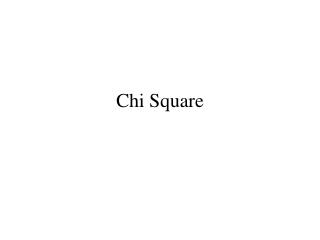DownloadDownload PresentationChi Square

# Chi Square

Download Presentation## Chi Square

- - - - - - - - - - - - - - - - - - - - - - - - - - - E N D - - - - - - - - - - - - - - - - - - - - - - - - - - -
##### Presentation Transcript

1. Chi Square

2. Chi Square • z, t and F tests are parametric (assume a normal distribution) • Chi square is non-parametric (distribution free) • A test of significance when data are expressed in frequencies or are expressed in percentages or proportions that can be reduced to frequencies. • Three uses: • To determine if a certain distribution differs from some predetermined theoretical distribution. • Testing hypotheses concerning the significance of the difference of the responses of two or more groups to stimuli. • Testing goodness of fit.

3. ( O - E ) 2 Chi Square x2 =  O 100 Flips of a coin Contingency table ( 40 - 50 ) 2 ( 60 - 50 ) 2 + = O E 50 50 40 50 Heads ( 10 ) 2 ( 10 ) 2 = + 60 50 Tails 50 50 100 100 100 100 = + 50 50 df = 1 = 2 + 2 = 4.00

4. Example 91 patients w/MI Treatment: Propranolol vs. Placebo Outcome: Survival > 28 days vs. Death < 28 days H0: treatment w/propranolol does not significantly influence the proportion of MI patients who survive at least 28 days. Ha: treatment w/propranolol does significantly influence the proportion of MI patients who survive at least 28 days.

5. ( O - E ) 2 x2 =  E Box 11–2 2 X 2 Contingency TableO = observed counts E = expected counts Outcome Survived > 28 days Death Propranolol (O) 38 33.13 7 11.87 45 45 Propranolol (E) Treatment 29 33.87 17 12.13 Placebo (O) 46 46 Placebo (E) 67 91 24 Row total E 1,1 = X Column total Study total

6. ( O - E ) 2 x2 =  E ( 7 – 11.87 ) 2 ( 29 – 33.87 ) 2 ( 17– 12.13 ) 2 ( 38 – 33.13 ) 2 = + + + 11.87 33.87 12.13 33.13 (4.87 ) 2 (4.87 ) 2 (4.87 ) 2 ( 4.87 ) 2 = + + + 11.87 33.87 12.13 33.13 23.72 23.72 23.72 23.72 = + + + 11.87 33.87 12.13 33.13 + + + = 0.72 2.00 0.70 1.96 = 5.38 df = (R – 1)(C – 1) = (2 –1)(2 – 1) = 1

7. [( O - E )-0.5] 2 x2 =  O Small numbers method (Yates correction for continuity) Box 11–2 2 X 2 Contingency TableO = observed counts E = expected counts Outcome Survived > 28 days Death Propranolol (O) 38 33.13 7 11.87 45 45 Propranolol (E) Treatment 29 33.87 17 12.13 Placebo (O) 46 46 Placebo (E) 67 91 24 Row total E 1,1 = X Column total Study total

8. [(O - E)-0.5] 2 x2 =  E [(38 – 33.13)-0.5] 2 [(7 – 11.87)-0.5] 2 [(29 – 33.87)-0.5] 2 [(17– 12.13)-0.5] 2 + = + + 12.13 11.87 33.87 33.13 [(4.87)-0.5] 2 [(4.87)-0.5] 2 [(4.87)-0.5] 2 [(4.87 )-0.5] 2 + = + + 12.13 11.87 33.87 33.13 19.09 19.09 19.09 19.09 + = + + 12.13 11.87 33.87 33.13 + + + 0.576 1.608 .563 1.573 = = 4.32 df = (R – 1)(C – 1) = (2 –1)(2 – 1) = 1

9. Chi Square in a large table O SA A NO D SD Group 1 54 Group 2 98 Group 3 48 70 44 26 26 34 200 E 54 Group 1 98 Group 2 48 Group 3 70 44 26 26 34 200 ( 48 – 34.3 ) 2 ( 12 –8.2 ) 2 ( 12 – 18.9 ) 2 +…. + + x2 =  34.3 8.2 18.9 df = (R – 1)(C – 1)

10. Mid-term review • Types of data • Normal distribution • Variance • Standard deviation and z scores • 2 X 2 table • Hypothesis testing H0: HA: • t-test • Pearson r/Linear regression • Chi square